×

Search anything:

# Learn to use Dask Dataframes

#### Software Engineering PythonGet this book -> Problems on Array: For Interviews and Competitive Programming

Reading time: 35 minutes | coding time: 10 minutes

Before we go on to work with Dask dataframes, we will revisit some of the basic topics like Pandas and Dask. We will understand how to use it with examples and when to use it and its limitations as well.

## What is Pandas?

Pandas is a python package used for data manipulation, analysis and cleaning. It is well suited for different kinds of data, such as:

• Tabular data with heterogeneously-typed columns
• Ordered and unordered time series data
• Arbitrary matrix data with row & column labels
• Unlabelled data

Dask is a parallel computing library in Python that scales the existing Python ecosystem. This python library can handle moderately large datasets on a single CPU by making use of multiple cores of machines or on a cluster of machines (distributed computing).

Dask stores the complete data on the disk in order to use less memory during computations. It uses data from the disk in chunks for processing. During processing, if intermediate values are generated they are discarded as soon as possible to save the memory consumption.

Also, it is not necessary that all the machines have same number of cores. Dask can internally handle the variations with the number of cores on a machine ie. it is possible that one system has 2 cores while the other has 4 cores.

A Dataframe is simply a two-dimensional data structure used to align data in a tabular form consisting of rows and columns.

A Dask DataFrame is composed of many smaller Pandas DataFrames that are split row-wise along the index. An operation on a single Dask DataFrame triggers many operations on the Pandas DataFrames that constitutes it. The pandas Dataframes may reside on the disk of a single machine or a number of different machines forming a cluster.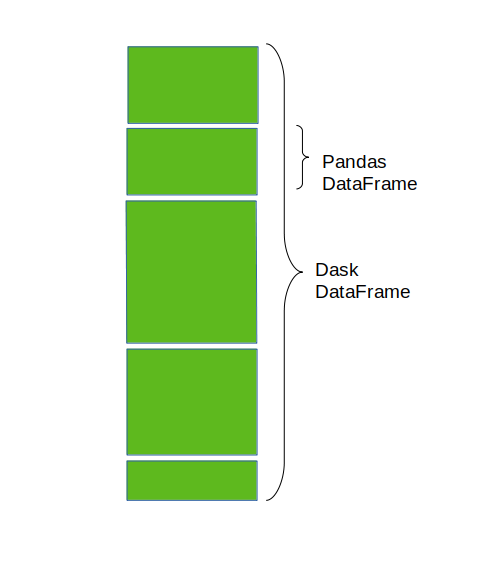When you are working with DataFrame operations that tend to reshape the data you must know that the rows are referred to as “axis 0” and the columns are referred to as “axis 1”. DataFrame operations by default work along axis 0.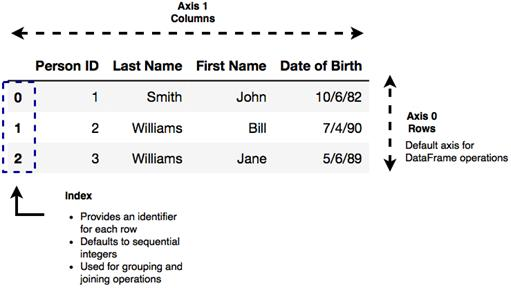## When to Use the Dask DataFrame?

Dask DataFrame is usually used when Pandas fails due to data size or computation speed. Pandas serves the purpose when you have tabular datasets that fit in memory. Dask becomes useful when the dataset that you want to analyze is larger than the RAM of your machine as it can run on your local computer or it can be scaled up to run on a cluster. It can also be also be used for long computations to accelarate the speed of computation by using many cores.

### Setup a Local Cluster

``````# import Client
client = Client()
client
``````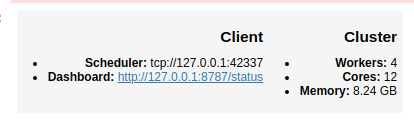By default, this sets up one worker per core. Click on the dashboard link to get helpful progress bars and see how your computation is going.

``````# import dask dataframe
df = dd.read_csv('path to csv file')
``````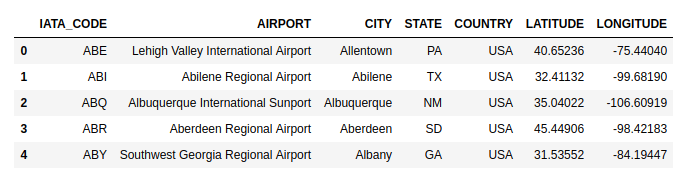head() only looks into the first partition and returns the output quickly. You should call compute() to get a full set of result When you call compute(), it returns a pandas dataframe.

### Memory Usage Tool

You can get to know if your data fits in memory. If yes, then you can just use regular pandas.

``````# memory usage tool
memory_usage = df.memory_usage(deep=True).compute()
# print in GB
memory_usage / 1e9
# total memory usage
memory_usage.sum() / 1e9
``````### Know the Columns in the Dataset

``````# display column names
df.columns
``````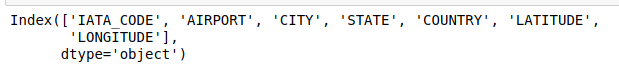### Select Specific Columns for Display

``````# select two columns

``````### Total Number of Rows

``````# print total number of rows
print(f'Number of rows: {len(df):,}.')
``````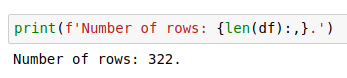### Total no of Partitions

``````# total no of partitions
df.npartitions
``````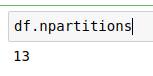### Get the First partition With get_partition

If you just want to quickly look at some data you can get the first partition with get_partition.

``````# get first partition
part_1= df.get_partition(1)
``````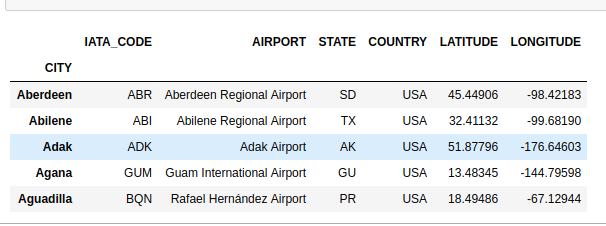### Get Distinct Elements

``````# all the distinct cities
``````### Row-wise Selections

``````# display number of rows with latitude >30
len(df[df.LATITUDE > 30])
````````````# display rows with latitude <30
df2=df[df.LATITUDE < 30]
``````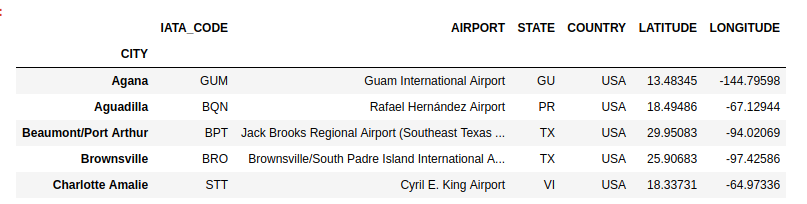### Set Index

``````# set CITY as index
df = df.set_index('CITY')
``````Data is sorted by the index column which allows for faster access, joins, groupby etc. However, sorting the data can be costly in parallel, so the index should be set when it is important and shold be done as infrequently as possible.

### Searching

``````# search a specfic city
df[df.CITY == 'Abilene'].compute()
``````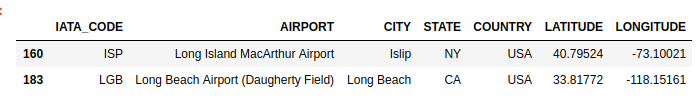``````# search in string
hs = df[df.AIRPORT.str.contains('Long')]
``````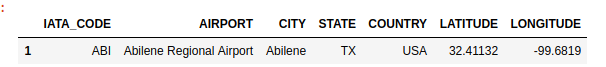### Groupby Operation

``````# group according to cities
frequency_city= df.groupby('CITY').size()
``````